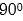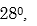##### Search### SECTION A (50 Marks)

1.

Solve by completing the square method3 marks

2.

Make K the subject of the formula.3 marks

3.

A varies as a constant and partly inversely as the cube root of P. When A=8, P=8 and when A=7, P=27.

Find the law connecting A and B, hence find A when P =125.

4 marks

4.

Find the percentage error in estimating the volume of a cone whose radius is 3.2cm and height is 8cm.

4 marks

5.

Evaluate without using Mathematical tables or calculator:2 marks

6.

Given that tandetermine tanleaving your answer in surd form of4 marks

7.

Determine the term independent of x in the expansion of2 marks

8.

Determine the values of x which satisfy the equation3 marks

9.

In the figure below, CF is a tangent to the circle, BC=3cm, ED=4cm and DC=2cm (not drawn to scale).Find

a) AB

b) FC

4 marks

10.

Find the values of x for which the matrix below is singular.3 marks

11.

The six interior angles of a hexagon form an arithmetic progression . If the smallest angle isFind the size of the largest angle of hexagon.

3 marks

12.

A coffee blender mixes grade A and B in the ratio 3:2 respectively. If grade A costs sh.30 per kg and grade B sh.25 per kg, at what price per kg should he sell the mixture in order to make a profit of 15% ?

3 marks

13.

Find the equation of a circle whose centre is (3, -2) and radius is 6 units.

3 marks

14.

A business premise value at Ksh 500,000 was appreciated at a rate of 2% and 3% during the first and second year respectively. Determine the value of the premise after the 2nd year.

2 marks

15.

The data below represents the ages in months at which 6 babies started walking; 10,12,13,14,12 and 11. Without using a calculator find the exact value of the variance.

3 marks

16.

The diagram below represents a rectangular prism in which AC=8cm, AB=10cm and <CAB=170 and length BE=30cm.4 marks

### SECTION B (50 Marks)

17.

Mang’oli’s Flying Company operates a flying service. It has two types of aeroplanes. The smaller one uses 180 litres of fuel per hour while the bigger one uses 300 litres per hour. The fuel available per week is 18000 litres. The company is allowed 80 flying hours per week. To keep the aeroplane in good condition the bigger aeroplane must be flown for x hours per week while smaller aeroplane must be flown for y hours per week.

a) Write down all the inequalities representing the above information.

b) On the grid provided at the end of this question paper, draw all the inequalities in (a) above by shading the unwanted regions.

c) The profit on the smaller aeroplane is sh4000 per hour while that on the bigger one is sh6000 per hour. Use the graph drawn in (b) above to determine the maximum profit that the company made per week.

10 marks

18.

a) Complete the table for the functions Y=3Sin 2x+and Y=Tan x given that10 marks

19.

In this question use a ruler and a pair of compasses only In the figure below, MN and CD are straight lines.Use the figure to;

i) Find a point R on MN such that R is equidistant from C and D.

ii) Complete a polygon CDRST with MN as its line of symmetry and hence measure the distance of R from TS.

b) Shade the region within the polygon in which a variable point X must lie given that X satisfies the following conditions:

I. X is nearer to CT than to CD.

II.RX is not more than 4.5cm.

III. <CXT >10 marks

20.

a) Given that the matrixfind B-1 the inverse of B.

b) Kweyu bought 200 bags of sugar and 300 bags of rice for a total of sh. 850,000. Beatrice bought 90 bags of sugar and 120 bags of rice for a total of sh.360,000. If the price of a bag of sugar is sh. x and that of rice is sh. y;

i) Form two equations to represent the information above.

ii) Use the matrix B-1 to find the prices of one bag of each item.

c) Kiplangat bought 225bags of sugar and 360 bags of rice. He was given a total discount of sh. 33,300. If the discount on the price of a bag of rice was 2%, calculate the percentage discount on the price of a bag of sugar.

10 marks

21.Find in terms of a and d.

i) BC:

ii) AX;

iii) DX.

b) Determine the values of h and k.

10 marks

22.

The table below shows monthly income tax rates for the year 2005.a) His monthly taxable income.

b) The monthly tax paid by Ole Sanguya.

10 marks

23.

A test is conducted for the purpose of employing a suitable typist. Their speeds of typing (words per minute) are shown:a) State the modal class.

b) Calculate the mean typing speed

c) What is the median typing speed?

d) In a company, the expenditure in a year was Sh.150,000 on transport, Sh.120,000 on wages and Sh. 25,000 on Sundry expenses. Represent this information on a pie- chart.

10 marks

24.

In the figure above, the two circles with centres M and N have radii 14cm and 7cm respectively. Given that the two circles intersect at X and Y and that <XMY=Find the area common to both circles.10 marks

Back Top